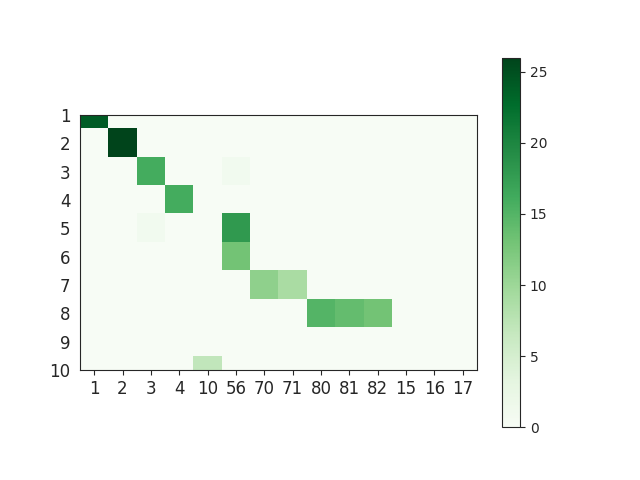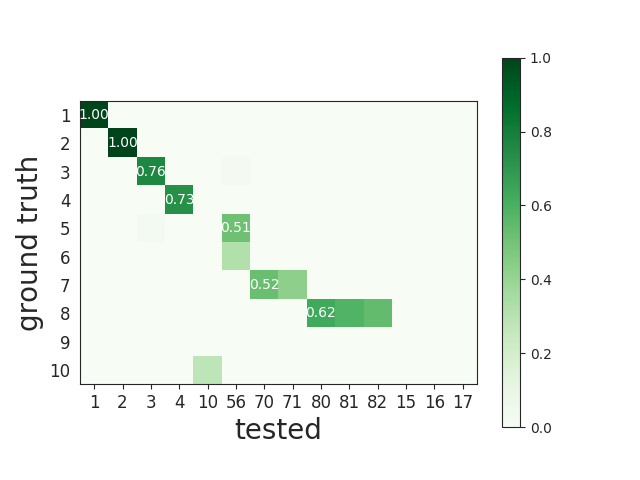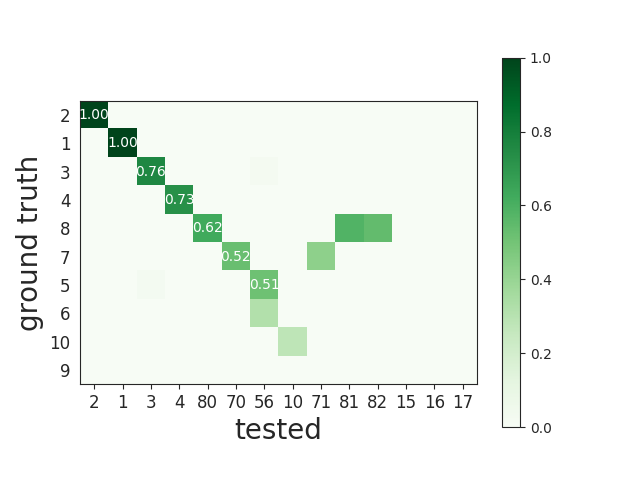# Spike sorting comparison methods¶

SpikeInterface has a comparison module that can be used for three distinct use cases:

1. compare a spike sorting output with a ground-truth dataset
2. compare the output of two spike sorters (symmetric comparison)
3. compare the output of multiple spike sorters

Even if the three comparison cases share the same underlying idea (they compare spike trains!) the internal implementations are slightly different.

## 1. Comparison with ground truth¶

A ground-truth dataset can be a paired recording, in which the a neuron is recorded both extracellularly and with a patch or juxtacellular electrode (either in vitro or in vivo), or it can be a simulated dataset (in silico) using spiking activity simulators such as MEArec.

The comparison to ground-truth datasets is useful to benchmark spike sorting algorithms.

As an example, the SpikeForest platform benchmarks the performance of several spike sorters on a variety of available gorund-truth datasets on a daily basis. For more detailse see spikeforest notes.

This is the main workflow used to compute perfomance metrics:

Given:
• i = 1, …, n_gt the list ground-truth (GT) units
• k = 1, …., n_tested the list of tested units from spike sorting output
• event_counts_GT[i] the number of spikes for each units of GT unit
• event_counts_ST[k] the number of spikes for each units of tested unit
1. Matching firing events

For all pairs of GT unit and tested unit we first count how many events are matched within a delta_time tolerence (0.4 ms by defualt).

This gives a matrix called match_event_count of size (n_gt X n_tested). This is an example of such matrices:Note that this matrix represents the number of true positive (TP) spikes of each pair. We can also compute the number of false negatives (FN) and false positive (FP) spikes.

• num_tp [i, k] = match_event_count[i, k]
• num_fn [i, k] = event_counts_GT[i] - match_event_count[i, k]
• num_fp [i, k] = event_counts_ST[k] - match_event_count[i, k]
1. Compute agreement score

Given the match_event_count we can then compute the agreement_score, which is normalized in the range [0, 1].

This is done as follows:

• agreement_score[i, k] = match_event_count[i, k] / (event_counts_GT[i] + event_counts_ST[k] - match_event_count[i, k])

which is equivalent to:

• agreement_score[i, k] = num_tp[i, k] / (num_tp[i, k] + num_fp[i, k] + num_fn[i,k])

or more practically:

• agreement_score[i, k] = intersection(I, K) / union(I, K)

which is also equivalent to the accuracy metric.

Here is an example of the agreement matrix, in which only scores > 0.5 are displayed:This matrix can be ordered for a better visualization:1. Match units

During this step, given the agreement_score matrix each GT units can be matched to a tested units. For matching, a minimum match_score is used (0.5 by default). If the agreement is below this threshold, the possible match is discarded.

There are two methods to perform the match: hungarian and best match.

The hungarian method finds the best association between GT and tested units. With this method, both GT and tested units can be matched only to another unit, or not matched at all.

For the best method, each GT unit is associated to a tested unit that has the best agreement_score, independently of all others units. Using this method several tested units can be associated to the same GT unit. Note that for the “best match” the minimum score is not the match_Score, but the chance_score (0.1 by default).

Here is an example of matching with the hungarian method. The first column represents the GT unit id and the second column the tested unit id. -1 means that the tested unit is not matched:

```GT    TESTED
0     49
1     -1
2     26
3     44
4     -1
5     35
6     -1
7     -1
8     42
...
```

Note that the SpikeForest project uses the best match method.

2. Compute performances

With the list of matched units we can compute performance metrics. Given : tp the number of true positive events, fp number of false positive event, fn the number of false negative event, num_gt the number of event of the matched tested units, the following metrics are computed for each GT unit:

• accuracy = tp / (tp + fn + fp)
• recall = tp / (tp + fn)
• precision = tp / (tp + fp)
• false_discovery_rate = fp / (tp + fp)
• miss_rate = fn / num_gt

The overall performances can be visualised with the confusion matrix, where the last columns counts FN and the last row counts FP.

• Hungarian:

Finds the best paring. If the matrix is square, then all units are associated. If the matrix is rectangular, then each row is matched. A GT unit (row) can be match one time only.

• Pros
• Each spike is counted only once
• Hit score near chance levels are set to zero
• Good FP estimation
• Cons
• Does not catch units that are split in several sub-units. Only the best math will be listed
• More complicated implementation
• Best

Each GT units is associated to the tested unit that has the best agreement score.

• Pros:
• Each GT unit is matched totally independently from others units
• The accuracy score of a GT unit is totally independent from other units
• It can identify over-merged units, as they would match multiple GT units
• Cons:
• A tested unit can be matched to multiple GT units, so some spikes can be counted several times
• FP scores for units associated several times can be biased
• Less robust with units having high firing rates

### Classification of identified units¶

Tested units are classified depending on their performance. We identify three different classes:

• well-detected units
• false positive units
• redundant units
• over-merged units

A well-detected unit is a unit whose performance is good. By default, a good performance is measured by an accuracy greater than 0.8-

A false positive unit has low agreement scores for all GT units and it is not matched.

A redundant unit has a relatively high agreement (>= 0.2 by default), but it is not a best match. This means that it could either be an oversplit unit or a duplicate unit.

An over-merged unit has a relatively high agreement (>= 0.2 by default) for more than one GT unit.

## 2. Compare the output of two spike sorters (symmetric comparison)¶

The comparison of two sorter is a quite similar to the procedure of compare to ground truth. The difference is that no assumption is done on which is the units are ground-truth.

So the procedure is the following:

• Matching firing events : same a ground truth comparison
• Compute agreement score : same a ground truth comparison
• Match units : only with hungarian method

As there is no ground-truth information, performance metrics are not computed. However, the confusion and agreement matrices can be visualised to assess the level of agreement.

## 3. Compare the output of multiple spike sorters¶

Comparison of multiple sorters uses the following procedure:

1. Perform pairwise symmetric comparisons between spike sorters
2. Construct a graph in which nodes are units and edges are the agreements between units (of different sorters)
3. Extract units in agreement between two or more spike sorters
4. Build agreement spike trains, which only contain the spikes in agreement for the comparison with the highest agreement score